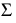Startup
This category depends on selecting the Unit Power Table method, and describes how the monetary cost associated with starting up or shutting down a unit (turbine) will be modeled. There are two methods in this category, one which does not model these costs (effectively assigning them a value of 0) and one which uses a table describing the combined costs for starting up and shutting down a unit.None
This is the default, do-nothing method.Unit Lumped Cost Method
For each Unit, this method lumps the cost of startup and shutdown into one value.
Slots Specific to This Method
Note:  Many of these slots have column or row dimensions based on the number of units. The rows/columns of these slots are expanded at the beginning of the run to match the value in the Number of Units slot. When first configuring this method, the user must enter the Number of Units, then run the model (stepping through one timestep is enough) to grow the slots to the right dimensions.
Unit Startup Cost Table
Type: TableSlot
Units: Value (\$)
Description: This table will indicate the cost of startup/shutdown of each unit.
Information: There will be one column for each unit and one row that represents the cost of startup/shutdown.
I/O: Required input
Unit Startup Cost
Type: AggSeriesSlot
Units: Value (\$)
Description: There is one column for each unit indicating the cost of startup/shutdown.
Information: In simulation, the value of Unit Startup Cost for each unit is the Unit Startup[u] * Unit Startup Cost Table [u].
I/O: Output only
Unit Startup
Type: AggSeriesSlot
Units: No Units
Description: A value of 1 indicates that the unit starts up at that date; otherwise the value is 0, indicating that the unit does not start up at that date.
Information: There is one column for each unit.
I/O: Output only
Unit Shutdown
Type: AggSeriesSlot
Units: No Units
Description: A value of 1 indicates that the unit shuts down at that date; otherwise the value is 0, indicating that the unit does not shut down at that date.
Information: There is one column for each unit.
I/O: Output only
Number Of Units Startup
Type: SeriesSlot
Units: No Units
Description: The number of units which start up at a given date. This value is the sum over the columns of Unit Startup.
Information:
I/O: Output only
Number Of Units Shutdown
Type: SeriesSlot
Units: No Units
Description: The number of units which shut down at a given date. This value is the sum over the columns of Unit Shutdown.
Information:
I/O: Output only
Plant Startup Cost
Type: SeriesSlot
Units: Value (\$)
Description: The total startup cost for the plant. This value is the sum over the columns of the Unit Startup Cost.
Information:
I/O: Output only
Method Details
In Simulation, if the Unit Lumped Cost method is selected, startup and shutdown will be summarized as follows:
• Calculate Unit Startup[t,u] = max(Unit Is Generating[t,u] - Unit Is Generating[t-1,u], 0)
• Calculate Unit Shutdown[t,u] = max(Unit Is Generating[t-1,u] - Unit Is Generating[t,u], 0)
• Calculate Number Of Units Startup[t] = sum(Unit Startup[t])
• Calculate Number Of Unit Shutdown[t] = sum(Unit Shutdown[t])
Note:  If the previous Unit Is Generating is not known, it is assumed that the unit is neither starting up or shutting down; Unit Startup and Unit Shutdown are set to zero. This may happens on the start timestep when the previous value is not known. Also, if the current Unit Is Generating is not valid, the method is exited without performing any computations or setting any slots.
This method will calculate the cost associated with startup/shutdown for each unit and the plant:
• Unit Startup Cost[t,u] = Unit Startup[t,u] * Unit Startup Cost Table[u]
• Plant Startup Cost[t] =(Unit Startup Cost[t])

Revised: 01/11/2023